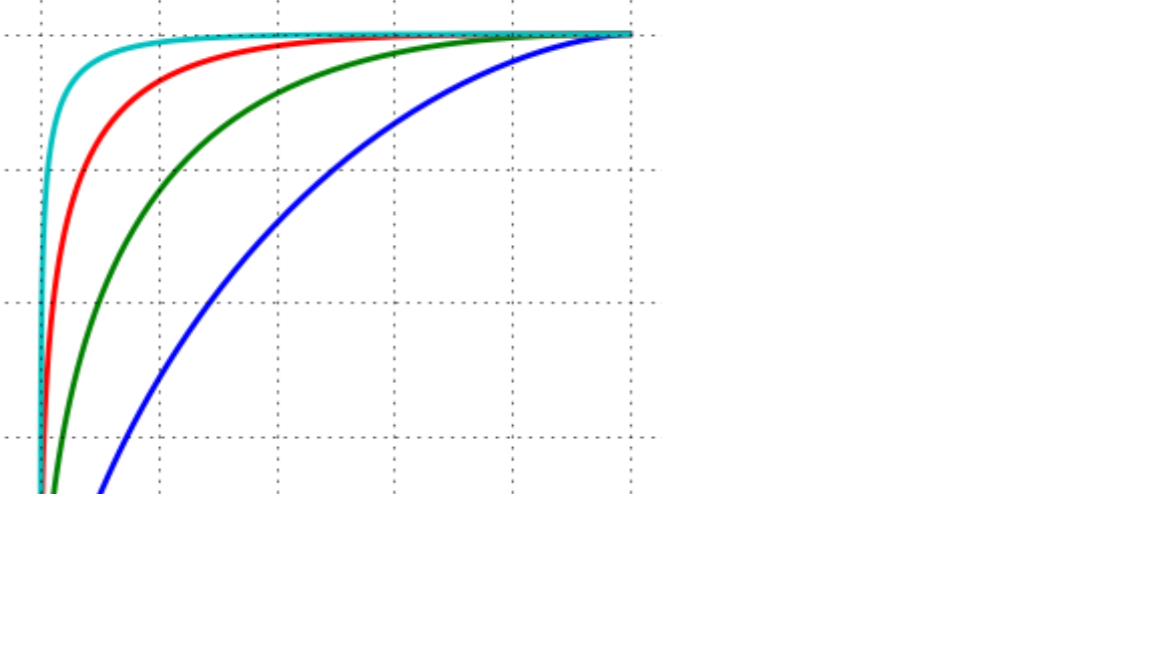## Introduction

### Furthermore, the coefficient of determination \$R^2\$ can be also calculated with respect to the AOC. Likewise the ROC curve, the shape of the REC curve can also be used as a guidance for the users to reveal additional information about the data modeling. The REC curve was implemented in Python.

``````# Loading Packages
import numpy as np
import matplotlib.pyplot as plt
import seaborn as sns
from sklearn.model_selection import cross_val_predict
from sklearn.metrics import r2_score
from sklearn import linear_model
from sklearn import datasets
from scipy.integrate import simps
``````
``````# Loading a sample regression dataset
X = boston.data
y_true = boston.target
``````
``````# Defining a simple linear regression model
LR = linear_model.LinearRegression()

# predicting using 10-folds cross-validation
y_pred = cross_val_predict(LR, X, y_true, cv=10)
``````
``````# Function for Regression Error Characteritic Curve

def REC(y_true , y_pred):

# initilizing the lists
Accuracy = []

# initializing the values for Epsilon
Begin_Range = 0
End_Range = 1.5
Interval_Size = 0.01

# List of epsilons
Epsilon = np.arange(Begin_Range , End_Range , Interval_Size)

# Main Loops
for i in range(len(Epsilon)):
count = 0.0
for j in range(len(y_true)):
if np.linalg.norm(y_true[j] - y_pred[j]) / np.sqrt( np.linalg.norm(y_true[j]) **2 + np.linalg.norm(y_pred[j])**2 ) < Epsilon[i]:
count = count + 1

Accuracy.append(count/len(y_true))

# Calculating Area Under Curve using Simpson's rule
AUC = simps(Accuracy , Epsilon ) / End_Range

# returning epsilon , accuracy , area under curve
return Epsilon , Accuracy , AUC

``````
``````# finding the deviation and accuracy, and area under curve for plotting
Deviation , Accuracy, AUC = REC(y_true , y_pred)
``````
``````# Calculating R^2 of the true and predicted values
RR = r2_score(y_true , y_pred)
``````
``````# Plotting
plt.figure(figsize=(14 , 8))

plt.subplot(1, 2, 1)
plt.scatter(y_true, y_pred,color = "darkorange")
plt.xlabel("Measured")
plt.ylabel("Predicted")
plt.plot([y_true.min(), y_true.max()], [y_true.min(), y_true.max()], 'k--', lw=4)
plt.text(45, -5, r"\$R^2 = %0.4f\$" %RR , fontsize=15)

plt.subplot(1, 2, 2)
plt.title("Regression Error Characteristic (REC)")
plt.plot(Deviation, Accuracy, "--b",lw =3)
plt.xlabel("Deviation")
plt.ylabel("Accuracy (%)")
plt.text(1.1, 0.07, "AUC = %0.4f" %AUC , fontsize=15)

plt.show()
``````